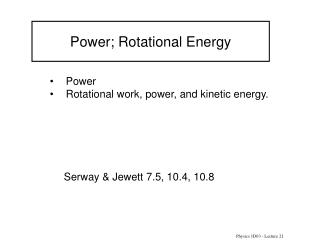# Power; Rotational Energy - PowerPoint PPT PresentationDownload PresentationPower; Rotational Energy

Power; Rotational EnergyDownload Presentation## Power; Rotational Energy

- - - - - - - - - - - - - - - - - - - - - - - - - - - E N D - - - - - - - - - - - - - - - - - - - - - - - - - - -
##### Presentation Transcript

1. Power; Rotational Energy • Power • Rotational work, power, and kinetic energy. Serway & Jewett 7.5, 10.4, 10.8 Physics 1D03 - Lecture 21

2. Recall: Physics 1D03 - Lecture 21

3. Power Power is the rate at which work is done: Average power = Work/time units: 1 J/s =1 watt (W) Instantaneous power: Average over an infinitesimal time dt, displacement ds; the work is dW = F • ds, and power is Physics 1D03 - Lecture 21

4. f F r ds = rdq d Rotational Work A bit of work, dW, is done in turning a nut through a tiny angle d : So, and so for a constant torque, Physics 1D03 - Lecture 21

5. Power: So, (again, angular velocity must be expressed in radians/second). Physics 1D03 - Lecture 21

6. Quiz A power screwdriver is intended to provide a torque of 0.5 N·m while turning at 120 revolutions per minute. The minimum power needed from the motor will be about • 60W • 6 W • 1 W Physics 1D03 - Lecture 21

7. vi w but  so K = ½ Iw 2 Kinetic energy of a rotating rigid body: Add up the kinetic energies of the particles: Physics 1D03 - Lecture 21

8. Quiz A wheel is spun up to speed by a motor that produces a constant power. It takes time t to reach an angular velocity . Assuming negligible friction at the axle, how long does it take to reach twice this angular velocity? Physics 1D03 - Lecture 21

9. Example A computer hard drive has four 100-gram platters (disks), 10 cm in diameter. (Uniform thin disk: I= ½ M R2) • How much kinetic energy do they have at 7200 rpm? • How long does a 7-watt motor take to get the drive up to speed? Physics 1D03 - Lecture 21

10. Example: Big Ben, a tower clock in London has an hour hand 2.7m long with a mass of 60kg and a minute hand 4.5m long with a mass of 100kg. Calculate the rotational kinetic energy of the two hands. (I=1/3 ML2) Big Ben Physics 1D03 - Lecture 21

11. Quiz A cone-shaped top is launched by winding a string of length L around the top, and pulling with a constant force F. How should the string be wound to do the greatest amount of work on the top? • wind it around the thick end • wind it around the thin end • it doesn’t matter how it is wound • not enough information F Physics 1D03 - Lecture 21

12. Summary • Power: P=dW/dt = F • v • Rotation: dW = t dq, P = t w, K = ½ Iw 2 • Suggested Problems:Chapter 7, problems 35, 40a (5910W) • Chapter 10, problems 21.(5th ed): • Chapter 7, problems 37, 47a • Chapter 10, problems 23. Physics 1D03 - Lecture 21